# probability-120611030603-phpapp02.pptx

15. Mar 2023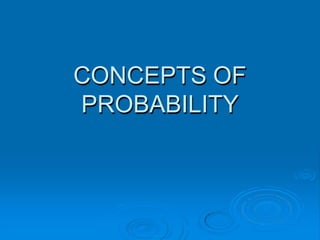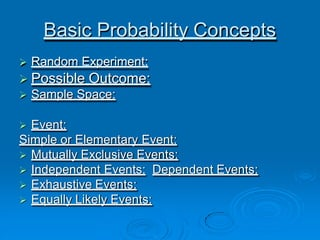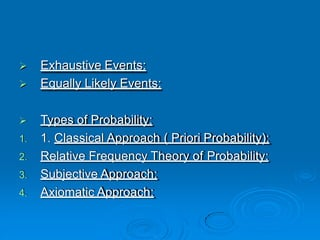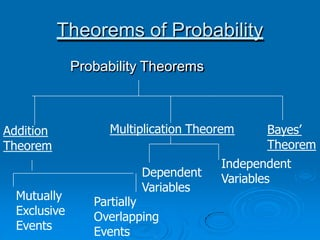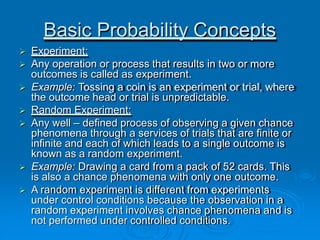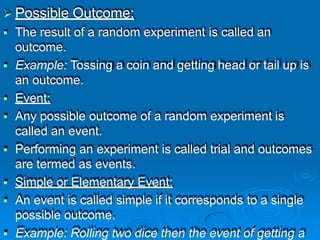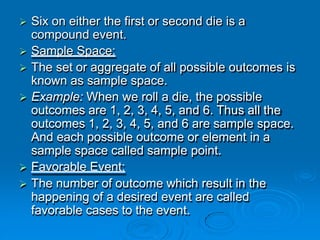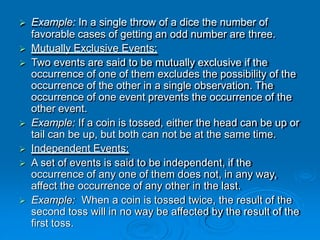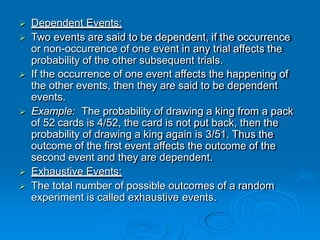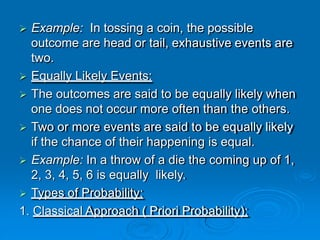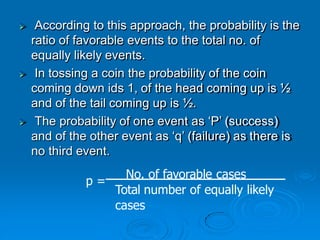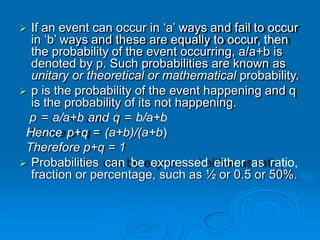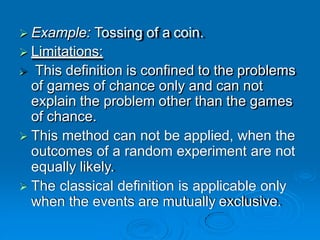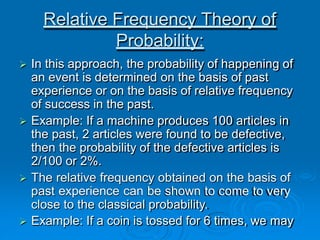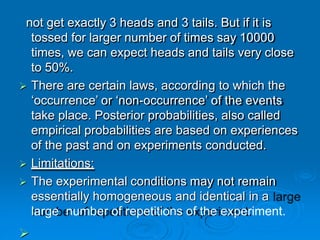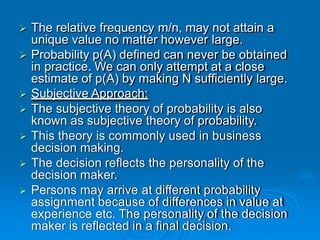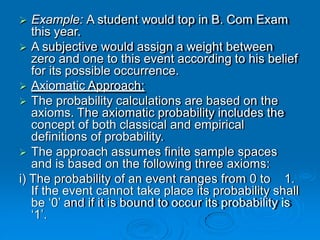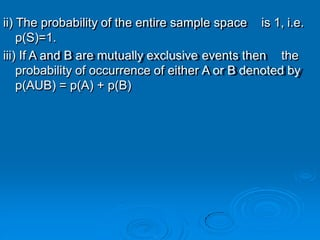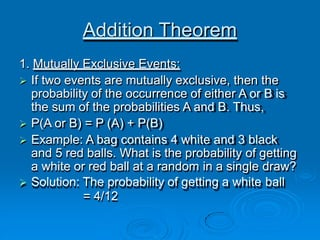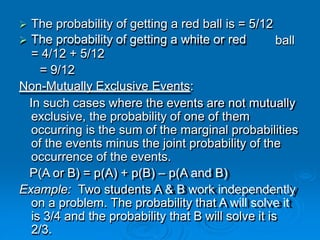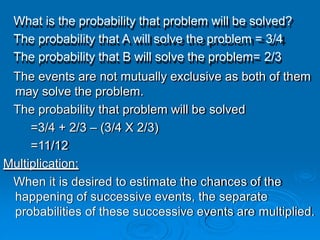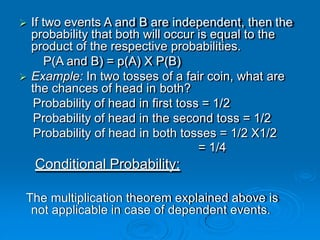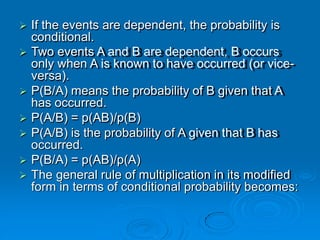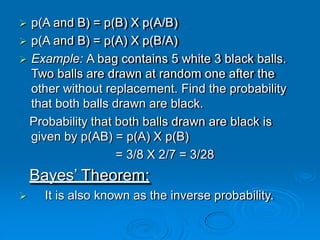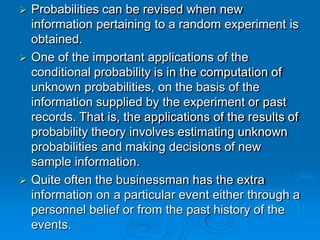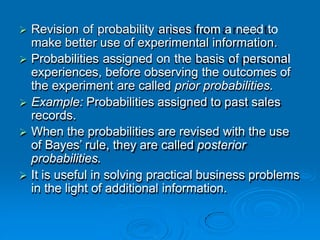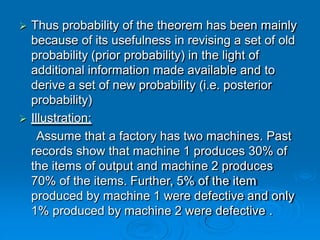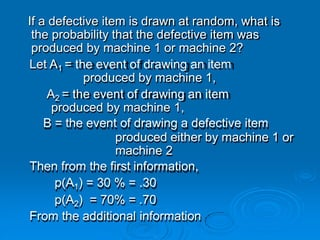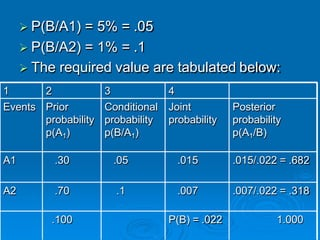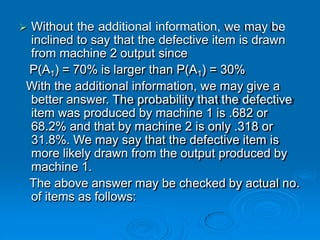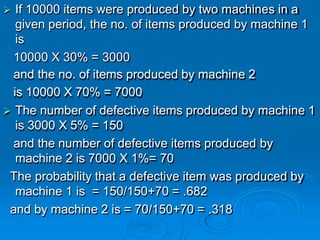1 von 32

### probability-120611030603-phpapp02.pptx

• 1. CONCEPTS OF PROBABILITY
• 2. Basic Probability Concepts  Random Experiment:  Possible Outcome:  Sample Space:  Event: Simple or Elementary Event:  Mutually Exclusive Events:  Independent Events: Dependent Events:  Exhaustive Events:  Equally Likely Events:
• 3.  Exhaustive Events:  Equally Likely Events:  Types of Probability: 1. 1. Classical Approach ( Priori Probability): 2. Relative Frequency Theory of Probability: 3. Subjective Approach: 4. Axiomatic Approach:
• 4. Theorems of Probability Probability Theorems Addition Theorem Multiplication Theorem Bayes’ Theorem Mutually Exclusive Events Partially Overlapping Events Dependent Variables Independent Variables
• 5. Basic Probability Concepts  Experiment:  Any operation or process that results in two or more outcomes is called as experiment.  Example: Tossing a coin is an experiment or trial, where the outcome head or trial is unpredictable.  Random Experiment:  Any well – defined process of observing a given chance phenomena through a services of trials that are finite or infinite and each of which leads to a single outcome is known as a random experiment.  Example: Drawing a card from a pack of 52 cards. This is also a chance phenomena with only one outcome.  A random experiment is different from experiments under control conditions because the observation in a random experiment involves chance phenomena and is not performed under controlled conditions.
• 6.  Possible Outcome:  The result of a random experiment is called an outcome.  Example: Tossing a coin and getting head or tail up is an outcome.  Event:  Any possible outcome of a random experiment is called an event.  Performing an experiment is called trial and outcomes are termed as events.  Simple or Elementary Event:  An event is called simple if it corresponds to a single possible outcome.  Example: Rolling two dice then the event of getting a
• 7.  Six on either the first or second die is a compound event.  Sample Space:  The set or aggregate of all possible outcomes is known as sample space.  Example: When we roll a die, the possible outcomes are 1, 2, 3, 4, 5, and 6. Thus all the outcomes 1, 2, 3, 4, 5, and 6 are sample space. And each possible outcome or element in a sample space called sample point.  Favorable Event:  The number of outcome which result in the happening of a desired event are called favorable cases to the event.
• 8.  Example: In a single throw of a dice the number of favorable cases of getting an odd number are three.  Mutually Exclusive Events:  Two events are said to be mutually exclusive if the occurrence of one of them excludes the possibility of the occurrence of the other in a single observation. The occurrence of one event prevents the occurrence of the other event.  Example: If a coin is tossed, either the head can be up or tail can be up, but both can not be at the same time.  Independent Events:  A set of events is said to be independent, if the occurrence of any one of them does not, in any way, affect the occurrence of any other in the last.  Example: When a coin is tossed twice, the result of the second toss will in no way be affected by the result of the first toss.
• 9.  Dependent Events:  Two events are said to be dependent, if the occurrence or non-occurrence of one event in any trial affects the probability of the other subsequent trials.  If the occurrence of one event affects the happening of the other events, then they are said to be dependent events.  Example: The probability of drawing a king from a pack of 52 cards is 4/52, the card is not put back, then the probability of drawing a king again is 3/51. Thus the outcome of the first event affects the outcome of the second event and they are dependent.  Exhaustive Events:  The total number of possible outcomes of a random experiment is called exhaustive events.
• 10.  Example: In tossing a coin, the possible outcome are head or tail, exhaustive events are two.  Equally Likely Events:  The outcomes are said to be equally likely when one does not occur more often than the others.  Two or more events are said to be equally likely if the chance of their happening is equal.  Example: In a throw of a die the coming up of 1, 2, 3, 4, 5, 6 is equally likely.  Types of Probability: 1. Classical Approach ( Priori Probability):
• 11.  According to this approach, the probability is the ratio of favorable events to the total no. of equally likely events.  In tossing a coin the probability of the coin coming down ids 1, of the head coming up is ½ and of the tail coming up is ½.  The probability of one event as ‘P’ (success) and of the other event as ‘q’ (failure) as there is no third event. p = No. of favorable cases Total number of equally likely cases
• 12.  If an event can occur in ‘a’ ways and fail to occur in ‘b’ ways and these are equally to occur, then the probability of the event occurring, a/a+b is denoted by p. Such probabilities are known as unitary or theoretical or mathematical probability.  p is the probability of the event happening and q is the probability of its not happening. p = a/a+b and q = b/a+b Hence p+q = (a+b)/(a+b) Therefore p+q = 1  Probabilities can be expressed either as ratio, fraction or percentage, such as ½ or 0.5 or 50%.
• 13.  Example: Tossing of a coin.  Limitations:  This definition is confined to the problems of games of chance only and can not explain the problem other than the games of chance.  This method can not be applied, when the outcomes of a random experiment are not equally likely.  The classical definition is applicable only when the events are mutually exclusive.
• 14. Relative Frequency Theory of Probability:  In this approach, the probability of happening of an event is determined on the basis of past experience or on the basis of relative frequency of success in the past.  Example: If a machine produces 100 articles in the past, 2 articles were found to be defective, then the probability of the defective articles is 2/100 or 2%.  The relative frequency obtained on the basis of past experience can be shown to come to very close to the classical probability.  Example: If a coin is tossed for 6 times, we may
• 15. not get exactly 3 heads and 3 tails. But if it is tossed for larger number of times say 10000 times, we can expect heads and tails very close to 50%.  There are certain laws, according to which the ‘occurrence’ or ‘non-occurrence’ of the events take place. Posterior probabilities, also called empirical probabilities are based on experiences of the past and on experiments conducted.  Limitations:  The experimental conditions may not remain essentially homogeneous and identical in a large number of repetitions of the experiment. 
• 16.  The relative frequency m/n, may not attain a unique value no matter however large.  Probability p(A) defined can never be obtained in practice. We can only attempt at a close estimate of p(A) by making N sufficiently large.  Subjective Approach:  The subjective theory of probability is also known as subjective theory of probability.  This theory is commonly used in business decision making.  The decision reflects the personality of the decision maker.  Persons may arrive at different probability assignment because of differences in value at experience etc. The personality of the decision maker is reflected in a final decision.
• 17.  Example: A student would top in B. Com Exam this year.  A subjective would assign a weight between zero and one to this event according to his belief for its possible occurrence.  Axiomatic Approach:  The probability calculations are based on the axioms. The axiomatic probability includes the concept of both classical and empirical definitions of probability.  The approach assumes finite sample spaces and is based on the following three axioms: i) The probability of an event ranges from 0 to 1. If the event cannot take place its probability shall be ‘0’ and if it is bound to occur its probability is ‘1’.
• 18. is 1, i.e. ii) The probability of the entire sample space p(S)=1. iii) If A and B are mutually exclusive events then the probability of occurrence of either A or B denoted by p(AUB) = p(A) + p(B)
• 19. Theorems of Probability Probability Theorems Addition Theorem Multiplication Theorem Bayes’ Theorem Mutually Exclusive Events Partially Overlapping Events Dependent Variables Independent Variables
• 20. Addition Theorem 1. Mutually Exclusive Events:  If two events are mutually exclusive, then the probability of the occurrence of either A or B is the sum of the probabilities A and B. Thus,  P(A or B) = P (A) + P(B)  Example: A bag contains 4 white and 3 black and 5 red balls. What is the probability of getting a white or red ball at a random in a single draw?  Solution: The probability of getting a white ball = 4/12
• 21. ball  The probability of getting a red ball is = 5/12  The probability of getting a white or red = 4/12 + 5/12 = 9/12 Non-Mutually Exclusive Events: In such cases where the events are not mutually exclusive, the probability of one of them occurring is the sum of the marginal probabilities of the events minus the joint probability of the occurrence of the events. P(A or B) = p(A) + p(B) – p(A and B) Example: Two students A & B work independently on a problem. The probability that A will solve it is 3/4 and the probability that B will solve it is 2/3.
• 22. What is the probability that problem will be solved? The probability that A will solve the problem = 3/4 The probability that B will solve the problem= 2/3 The events are not mutually exclusive as both of them may solve the problem. The probability that problem will be solved =3/4 + 2/3 – (3/4 X 2/3) =11/12 Multiplication: When it is desired to estimate the chances of the happening of successive events, the separate probabilities of these successive events are multiplied.
• 23.  If two events A and B are independent, then the probability that both will occur is equal to the product of the respective probabilities. P(A and B) = p(A) X P(B)  Example: In two tosses of a fair coin, what are the chances of head in both? Probability of head in first toss = 1/2 Probability of head in the second toss = 1/2 Probability of head in both tosses = 1/2 X1/2 = 1/4 Conditional Probability: The multiplication theorem explained above is not applicable in case of dependent events.
• 24.  If the events are dependent, the probability is conditional.  Two events A and B are dependent, B occurs only when A is known to have occurred (or vice- versa).  P(B/A) means the probability of B given that A has occurred.  P(A/B) = p(AB)/p(B)  P(A/B) is the probability of A given that B has occurred.  P(B/A) = p(AB)/p(A)  The general rule of multiplication in its modified form in terms of conditional probability becomes:
• 25.  p(A and B) = p(B) X p(A/B)  p(A and B) = p(A) X p(B/A)  Example: A bag contains 5 white 3 black balls. Two balls are drawn at random one after the other without replacement. Find the probability that both balls drawn are black. Probability that both balls drawn are black is given by p(AB) = p(A) X p(B) = 3/8 X 2/7 = 3/28 Bayes’ Theorem:  It is also known as the inverse probability.
• 26.  Probabilities can be revised when new information pertaining to a random experiment is obtained.  One of the important applications of the conditional probability is in the computation of unknown probabilities, on the basis of the information supplied by the experiment or past records. That is, the applications of the results of probability theory involves estimating unknown probabilities and making decisions of new sample information.  Quite often the businessman has the extra information on a particular event either through a personnel belief or from the past history of the events.
• 27.  Revision of probability arises from a need to make better use of experimental information.  Probabilities assigned on the basis of personal experiences, before observing the outcomes of the experiment are called prior probabilities.  Example: Probabilities assigned to past sales records.  When the probabilities are revised with the use of Bayes’ rule, they are called posterior probabilities.  It is useful in solving practical business problems in the light of additional information.
• 28.  Thus probability of the theorem has been mainly because of its usefulness in revising a set of old probability (prior probability) in the light of additional information made available and to derive a set of new probability (i.e. posterior probability)  Illustration: Assume that a factory has two machines. Past records show that machine 1 produces 30% of the items of output and machine 2 produces 70% of the items. Further, 5% of the item produced by machine 1 were defective and only 1% produced by machine 2 were defective .
• 29. If a defective item is drawn at random, what is the probability that the defective item was produced by machine 1 or machine 2? Let A1 = the event of drawing an item produced by machine 1, A2 = the event of drawing an item produced by machine 1, B = the event of drawing a defective item produced either by machine 1 or machine 2 Then from the first information, p(A1) = 30 % = .30 p(A2) = 70% = .70 From the additional information
• 30.  P(B/A1) = 5% = .05  P(B/A2) = 1% = .1 1  The required value are tabulated below: 2 3 4 Events Prior probability p(A1) Conditional probability p(B/A1) Joint probability Posterior probability p(A1/B) A1 .30 .05 .015 .015/.022 = .682 A2 .70 .1 .007 .007/.022 = .318 .100 P(B) = .022 1.000
• 31.  Without the additional information, we may be inclined to say that the defective item is drawn from machine 2 output since P(A1) = 70% is larger than P(A1) = 30% With the additional information, we may give a better answer. The probability that the defective item was produced by machine 1 is .682 or 68.2% and that by machine 2 is only .318 or 31.8%. We may say that the defective item is more likely drawn from the output produced by machine 1. The above answer may be checked by actual no. of items as follows:
• 32.  If 10000 items were produced by two machines in a given period, the no. of items produced by machine 1 is 10000 X 30% = 3000 and the no. of items produced by machine 2 is 10000 X 70% = 7000  The number of defective items produced by machine 1 is 3000 X 5% = 150 and the number of defective items produced by machine 2 is 7000 X 1%= 70 The probability that a defective item was produced by machine 1 is = 150/150+70 = .682 and by machine 2 is = 70/150+70 = .318